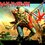# The knapsack cryptosystem at work

This note is an example of the knapsack crypto-system at work.So before you got through this note I recommend reading the main one . Click here

Suppose that a typical user of this crypto-system selects as the secret key the super-increasing sequence ${3,5,11,20,41}$ and the modulus $m = 85$ and the multiplier $a = 44$.Each member of the super-increasing sequence is multiplied by 44 and reduced modulo 85 to yield ${47,50,59,30,19}$.This is the encryption key the user submit to the public directory.

Suppose someone wants to send a plaintext message to the user such as "HELP US". First we convert it into a string of 1's and 0's $M = 00111 00100 01011 10100 10010$ The string is then broken up into blocks of digits,in the current case,blocks of length 5.Using the listed public keys to encrypt,the sender transforms the successive blocks into $47*0+50*0+59*1+30*1+19*1 = 108$ $47*0+50*0+59*1+30*0+19*0 = 59$ $47*0+50*1+59*0+30*1+19*1 = 99$ $47*0+50*1+59*1+30*1+19*1 = 158$ $47*1+50*0+59*1+30*0+19*0 = 106$ $47*1+50*0+59*0+30*1+19*0 = 77$

The transmitted ciphertext consists of the following series of positive integers ${108,59,99,158,106,777}$.

To read the message the legitimate receiver first solves the congruence $44x \equiv 1 \pmod{85}$,yielding $y \equiv 29 \pmod{85}$.Then each ciphertext number is multiplied by 29 and reduced modulo 85 to produce a super-increassing knapsack problem.

For example,$108$ is converted to $72$ as $108*29 \equiv 72 \pmod{85}$ and the corresponding knapsack problem is $3x_1 + 5x_2 + 11x_3 + 20x_4+41x_5 = 72$.

The procedure for solving super-increasing knapsack problems quickly yields the solution $x_1 = x_2 =0$,$x_3 = x_4=x_5 = 1$,in this way $00111$,the first block of the binary equivalent of the plaintext is obtained.7 years, 3 months ago

This discussion board is a place to discuss our Daily Challenges and the math and science related to those challenges. Explanations are more than just a solution — they should explain the steps and thinking strategies that you used to obtain the solution. Comments should further the discussion of math and science.

When posting on Brilliant:

• Use the emojis to react to an explanation, whether you're congratulating a job well done , or just really confused .
• Ask specific questions about the challenge or the steps in somebody's explanation. Well-posed questions can add a lot to the discussion, but posting "I don't understand!" doesn't help anyone.
• Try to contribute something new to the discussion, whether it is an extension, generalization or other idea related to the challenge.

MarkdownAppears as
*italics* or _italics_ italics
**bold** or __bold__ bold
- bulleted- list
• bulleted
• list
1. numbered2. list
1. numbered
2. list
Note: you must add a full line of space before and after lists for them to show up correctly
paragraph 1paragraph 2

paragraph 1

paragraph 2

[example link](https://brilliant.org)example link
> This is a quote
This is a quote
    # I indented these lines
# 4 spaces, and now they show
# up as a code block.

print "hello world"
# I indented these lines
# 4 spaces, and now they show
# up as a code block.

print "hello world"
MathAppears as
Remember to wrap math in $$ ... $$ or $ ... $ to ensure proper formatting.
2 \times 3 $2 \times 3$
2^{34} $2^{34}$
a_{i-1} $a_{i-1}$
\frac{2}{3} $\frac{2}{3}$
\sqrt{2} $\sqrt{2}$
\sum_{i=1}^3 $\sum_{i=1}^3$
\sin \theta $\sin \theta$
\boxed{123} $\boxed{123}$

Sort by:

How is the message' calculated? How "HELP US" is converted to given binary? I didn't get it. Please explain.

- 3 years, 2 months ago